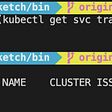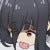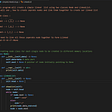## [LeetCode][python3]0031. Next Permutation

1. My Solution
`class Solution:    def nextPermutation(self, nums: List[int]) -> None:        """        Do not return anything, modify nums in-place instead.        """        if len(nums)<=1:            return        rec=0        for i in range(len(nums)-2,-1,-1):            if nums[i]<nums[i+1]:                rec=i                break        swap=i        for i in range(len(nums)-1,rec,-1):            if nums[i]>nums[rec]:                swap=i                break        if rec==swap:            nums.sort()            return        # print(rec,swap)        nums[rec]^=nums[swap]        nums[swap]^=nums[rec]        nums[rec]^=nums[swap]                rec+=1        swap=len(nums)-1        while rec<swap:            nums[rec]^=nums[swap]            nums[swap]^=nums[rec]            nums[rec]^=nums[swap]            rec+=1            swap-=1`
`class Solution:    def nextPermutation(self, nums: List[int]) -> None:        """        Do not return anything, modify nums in-place instead.        """        # from back to front, find the first descending num        # swap the num with the smallest num larger than it from back        # reverse all nums in the second half of the list        for i in range(len(nums)-1,-1,-1):            if i != 0:                # first descending num                if nums[i-1] < nums[i]:                    break        # entire list in descending order        if i == 0:            nums.reverse()            return        # one num to be swapped        k = i - 1                # the other num to be swapped        for j in range(len(nums)-1,-1,-1):            if nums[j] > nums[k]:                break              # swap the numbers        nums[j], nums[k] = nums[k], nums[j]        # reverse the second portion of the list        left, right = k+1, len(nums)-1        while left < right:            nums[left], nums[right] = nums[right], nums[left]            left += 1            right -= 1`

--

--

--

## More from N2I

HI I’m N2I. Now a SWE in Taiwan. Check out more about me in https://nzi2020.blogspot.com/ or contact via email: nayzi9999@gmail.com

Love podcasts or audiobooks? Learn on the go with our new app.

## Le Wagon is not only about coding skills## Why is Python the Best-Suited Programming Language## Introducing CodeCast Series ✨## Diving into Kotlin DSL Part-2: Kotlin enters the Gradle realm## Game Dev Log (2)## Canary deployments: Go easy with Ketch 0.2!## Platform vs. PlatformTarget in any .NET build.## N2I

HI I’m N2I. Now a SWE in Taiwan. Check out more about me in https://nzi2020.blogspot.com/ or contact via email: nayzi9999@gmail.com

## More from Medium